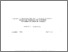# A Quadratic Approximation Method Based on Augmented Lagrangian Functions for Nonconvex Nonlinear Programming Problems

Wierzbicki, A.P. (1978). A Quadratic Approximation Method Based on Augmented Lagrangian Functions for Nonconvex Nonlinear Programming Problems. IIASA Working Paper. IIASA, Laxenburg, Austria: WP-78-061Preview Text WP-78-061.pdf Download (1MB) | Preview

## Abstract

The paper describes an algorithm for solving nonlinear programming problems of fairly general type, in particular nonconvex problems that can be convexified via augmented Lagrarlgian functions. The algorithm consists of two phases. In the first phase, a point in a rather large neighborhood of an optimal solution is crudely but effectively estimated by a shifted-increased penalty function algorithm. In the second phase, a saddle point of an augmented Lagrangian function and thus an optimal solution together with corresponding Lagrange multipliers are found by a rapidly convergent method of successive quadratic approximations. The switch between these two phases is adaptive. According to the existing experience in nonlinear programming algorithms, the proposed algorithm combines the best local effectiveness of a quadratic approximation method with the global robustness of a penalty method; due to the convexifying properties of augmented Lagrangian functions, the algorithm can solve nonconvex problems which satisfy second-order sufficient conditions of optimality at the solution.

For the sake of a clear presentation, a short review of some basic facts in the theory of Lagrangian functions, quadratic approximations, penalty functions and augmented Lagrangian functions is given in the first part of the paper. Then the quadratic approximations for augmented Lagrangeans are discussed in detail, in particular, in the case when these functions are not twice differentiable which corresponds to the lack of strict complementarity at the optimal solution. The double-phase algorithm is presented and commented upon. The proofs of convergence of the algorithm are given.

Item Type: Monograph (IIASA Working Paper) System and Decision Sciences - Core (SDS) IIASA Import 15 Jan 2016 01:44 27 Aug 2021 17:08 https://pure.iiasa.ac.at/857View Item